# Divide

Divide substance 110 cm long to two parts so that it first part is 10 cm longer than the second part and one part will be 10 times longer than the second portion. How long the parts will be?

Correct result:

a =  60 cm
b =  50 cm

#### Solution:

a+b=110
a = 10+b

a+b = 110
a-b = 10

a = 60
b = 50

Our linear equations calculator calculates it.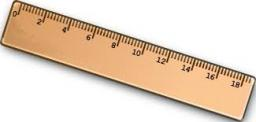We would be pleased if you find an error in the word problem, spelling mistakes, or inaccuracies and send it to us. Thank you!Tips to related online calculators
Do you have a linear equation or system of equations and looking for its solution? Or do you have quadratic equation?
Do you want to convert length units?

## Next similar math problems:

• Dividing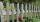Divide the three line segment 13 cm, 26 cm and 19.5 cm long for parts so that the individual parts were equally long and longest. How long will the individual parts and how many it will?
• Tablecloth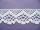The table top is 90 cm long and 50 cm wide. How many cm lace edging is necessary for the tablecloth that hangs on each side by 10 cm?
• PegsFrom two sticks 240 cm and 210 cm long, it is necessary to cut the longest possible pegs for flowers so that no residues remain. How many pegs will it be?
• Buns and toastsTwo buns weigh 10 grams more than two toasts. One Bun and two Toast weigh a total of 110 grams. How many grams weigh three toasts? How many grams does a Bun weigh?
• Number of songs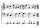Write an expression for the number of songs they need for this show. Evan and Peter have a radio show that has 2 parts. They need 4 fewer than 11 songs in the first part. In the second part, they need 5 fewer than 3 times the number of songs in the first
• GardensThe garden has the square shape with circumference 124 m. Divide it into two rectangular gardens, one should circumference 10 meters more than the second. What size will have a gardens?
• Clotheslines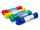Mommy needs 6 clothesline 360 cm long. Clotheslines is sold in the length of eight meters. How many of these clotheslines must buy mom if any does not want to be connected? How much mommy pay for the clotheslines when 1 piece costs 24kč?Add marks (+, -, *, /, brackets) to fullfill equations 1 3 6 5 = 10 This is for the 4th grade of the primary school - with no negative numbers yet
• My fatherMy father cut 78 slats on the fence. The shortest of them was 97 cm long, the longer one was 102 cm long. What was the total length of the slats in cm?
• I think numberI think number.When I add 841 to it and subtract 157, I get a number that is 22 greater than 996. What number I thinking?
• RemaindersIt is given a set of numbers { 170; 244; 299; 333; 351; 391; 423; 644 }. Divide this numbers by number 66 and determine set of remainders. As result write sum of this remainders.
• Chocolate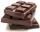How many times should be broken chocolate consisting of 10 × 12 pieces to get the 120 parts?
• Circles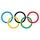For the circle c1(S1; r1=146 cm) and c2(S2; r2 = 144 cm) is distance of centers |S1S2| = 295 cm. Determine the distance between the circles.
• Pump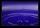From the pump in the yard dripping water. Every drop is 1 ml. How many drops will be necessary before filling a bucket with a volume of 10 l? Calculate how long it will fulfill the bucket when drops fall each second.
• The rodThe rod has a length of 90cm. Half is painted blue, the third is red and the rest is yellow. How long is the yellow part of the rod?
• Birds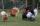On the farm they have a total of 110 birds. Geese and turkeys together is 47. Hens is three times more than the turkey. How much is poultry by species?
• The thirdThe one-third rod is blue, one-half of the rod is red, the rest of the rod is white and measures 8 cm. How long is the whole rod?